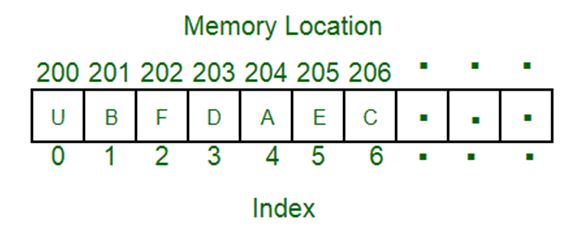# Python program to find the occurrence of a particular number in an array

Here, we are going to learn how to find the occurrence of a particular number in an array using python program?
Submitted by Suryaveer Singh, on June 08, 2019

In this article, we would learn about arrays and how to code for an array question asked? So starting off we would first learn about arrays i.e. what an array is?

ARRAY: An array can be defined as a container object that is used to hold a fixed number of values of a single type of data. The main purpose of an array is to store multiple items of the same type together.This is the simple idea that you need to understand about the array, now starting with the simple question.

Question:

Suppose you are given with an array that contains ints. Your task is to return the number of 3 in the array.

Example:

```    Count3([1, 2, 3]) = 1
Count3([1, 3, 3]) = 2
Count9([1, 3, 9, 3, 3]) = 3
```

Solution:

Here we have to again consider a variable which is initially equal to zero to keep the count of a number of 3 and also our function is only defined for nums as our array would only contain integers as mentioned earlier.

Code:

```def Count3(nums):
count = 0
for num in nums:
if num == 3:
count = count + 1

return count

print (Count3([1,2,3,4,3,3,3,]))
```

Output

```4
```

Preparation